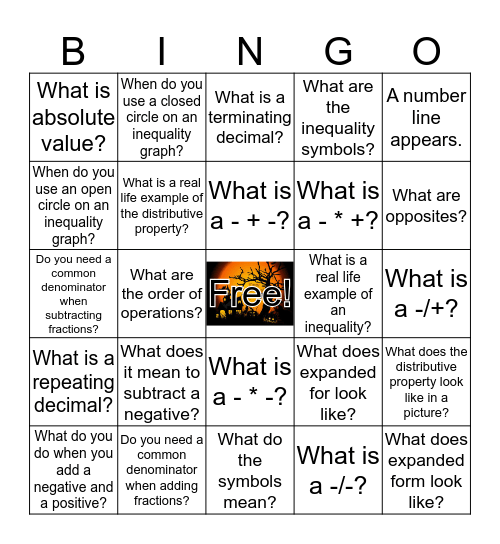# ATN Presentation BingoThis bingo card has a free space and 24 words: What does it mean to subtract a negative?, Do you need a common denominator when adding fractions?, What is a - + -?, What do you do when you add a negative and a positive?, A number line appears., What is absolute value?, When do you use an open circle on an inequality graph?, When do you use a closed circle on an inequality graph?, What is a real life example of an inequality?, What is a - * -?, What is a - * +?, Do you need a common denominator when subtracting fractions?, What does expanded for look like?, What does the distributive property look like in a picture?, What is a real life example of the distributive property?, What is a -/-?, What is a -/+?, What is a terminating decimal?, What are opposites?, What are the order of operations?, What does expanded form look like?, What is a repeating decimal?, What are the inequality symbols? and What do the symbols mean?.

## Play Online